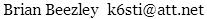Measuring Noise Figure without a Noise Source

Noise factor is the ratio of noise power to the irreducible power of thermal noise in a system or component. It is a measure of excess noise. A component that adds no noise has a noise factor of 1. One that doubles thermal noise power has a noise factor of 2. Noise figure is noise factor expressed in dB.

I wanted to measure the noise figure of a cable-TV preamp in the FM broadcast band. I didn't have a calibrated noise source so I found a way to use calibrated signal generators and a spectrum analyzer instead.

Method

First I calibrate the spectrum analyzer with the signal generators. Then I use it to measure the preamp output noise level with its input terminated. I subtract the power due to thermal noise to obtain the system noise figure, which includes noise from the preamp and the spectrum analyzer. Finally, I measure the noise figure of the spectrum analyzer alone and then calculate the preamp noise figure.

To lower the noise figure of my HP 141T/8553B/8552B spectrum analyzer, I precede it with an HP 461A amplifier for all measurements.

My absolute level reference is a calibrated HP 8640B signal generator. My relative level reference is a calibrated HP 3336C level generator. Instead of the 141T display scale, I use the 3336C to set the relative levels of the 8640B signal and the noise. Correction factors account for the the noise bandwidth of the 8552B IF filter and the response of its logarithmic envelope detector to Gaussian noise.

First I tune the 3336C and 141T to 1 MHz. I set the 141T to nonscanning mode and 2 dB/div. Using the very accurate 3336C attenuator, I establish two levels 10 dB apart. I connect an HP 3456A digital voltmeter to the 8552B vertical output and record the corresponding DC voltages. This method provides much better accuracy and repeatability than using the screen calibration. I use maximum 8552B video filtering and engage the 3456A noise filter. I position the 0 dB level at the top of the screen and the −10 dB level occurs about one division below midscreen. I believe these levels keep the log detector from clipping or bottoming out on noise extremes.

Next I tune the 141T to the frequency of interest in the FM broadcast band and terminate the system input. I set the 8552B IF bandwidth to 100 kHz and adjust the log reference level so that the vertical output voltage corresponds to the −10 dB level. I replace the termination with the 8640B and tune it to peak the spectrum analyzer response. Then I adjust its output level until the vertical output voltage corresponds to the 0 dB level. Call this signal level L.

As described in HP Application Note 1303, a spectrum analyzer's logarithmic envelope detector undermeasures Gaussian noise by 2.51 dB. Its IF filter overmeasures because its noise bandwidth exceeds its −3 dB bandwidth. I measured the response of my 100 kHz filter and calculated its noise bandwidth as 107.9 kHz, a 0.33 dB overmeasurement. Thus a 10 dB difference for two CW signals becomes a difference of 10 − 2.51 + 0.33 = 7.8 dB for a CW signal and noise. Therefore the absolute noise level is L − 7.8. (L includes noise, but as the app note shows in Fig. G, the error is vanishingly small for a log detector when the noise is more than a few dB down.)

Thermal noise power at room temperature is −174 dBm/Hz. In a 100 kHz bandwidth it becomes −174 + 10log(100000) = −124 dBm. Therefore the noise figure is L − 7.8 − −124 = L + 116.2 dB.

From HP Application Note 57-1,

F = F1 + (F2 - 1) / G1

where F is the noise factor of a cascaded system, F1 is the noise factor of the first stage, G1 is its power gain, and F2 is the noise factor of the rest of the system.

Therefore

P = 10log[10S/10 - (10R/10 - 1) / 10G/10]

where P is preamp noise figure, S is system noise figure, R is the 461A + 141T noise figure, and G is preamp gain, all in dB.

Example

I measured the preamp gain as 13.7 dB at 96.9 MHz using the 8640B and a Wavetek SAM signal meter. 3336C signal levels 10 dB apart yielded 8552B vertical outputs of −0.763 V (0 dB) and −0.278 V (−10 dB) on the 3456A voltmeter. After adjusting the 8552B reference level so that the vertical output was −0.278 V with the 461A input terminated in 50Ω, the 8640B signal required for −0.763 V was −103.7 dBm. This implies a 461A + 141T noise figure of 116.2 − 103.7 = 12.5 dB. Adding the preamp and readjusting the reference level for −0.278 V with the preamp input terminated in 75Ω, the signal required for −0.763 V was −110.5 dbm. This yields a system noise figure of 116.2 − 110.5 = 5.7 dB. Plugging these numbers into the second equation yields a preamp noise figure of 4.8 dB. I accounted for the losses of the 50:75Ω matching networks and test cables.

June 3, 201188–108 MHz